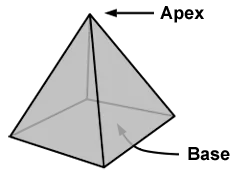# Square Pyramid Volume Calculator

This Square Pyramid Volume Calculator is designed to help you determine the volume of a square pyramid.

To use the calculator, simply enter the base edge and height of the square pyramid and click "Calculate". As you enter new dimensions into the volume calculator, a running total will also be displayed.

## Basic definition and general information about the Pyramid

A pyramid is a three-dimensional solid geometric figure that usually has a square or triangular base and sloping sides that meet in a point. The highest point where all the sides meet together is referred to as the apex.The following formula is used to calculate the volume of a square pyramid:

• (Area of Base ÷ 3) x Height [ It is crucial to understand that the base is in the form of a square, thus, the area is represented by the length of one side squared - L2 ]

Note: Units are provided for convenience purposes only and do not affect the calculations.

Units used for measuring the volumes of solid objects are as follows:

• cubic feet
• cubic meters
• cubic yards

Units used to measure the volumes of liquids are:

• liters
• quarts
• pints
• gallons